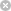RTXI  2.1RlsFilter Class Reference

#include <rls_filt.h>

Inheritance diagram for RlsFilter:Public Member Functions

RlsFilter (int num_taps, double *coeff, double delta, double lambda, logical quan_enab, long coeff_quan_factor, long input_quan_factor, int tap_for_trans, int secondary_tap, int transient_len)

double UpdateTaps (double true_samp, double estim_samp, logical trans_save_enab)

void ResetTaps (void)Public Member Functions inherited from AdaptiveFir
AdaptiveFir (int num_taps, double *coeff, logical quan_enab, long coeff_quan_factor, long input_quan_factor, int tap_for_trans, int secondary_tap, int transient_len)

void DumpAvgTransient (int decim_factor)

void DumpTransient (int decim_factor)

void DumpAvgTrajectory (double min_dist)

void DumpTrajectory (double min_dist)

void GetTaps (double *taps)Public Member Functions inherited from DirectFormFir
DirectFormFir (int num_taps, double *coeff, logical quan_enab, long coeff_quan_factor, long input_quan_factor)

double ProcessSample (double input_val)

long ProcessSample (long input_val)

int GetNumTaps (void)Public Member Functions inherited from FilterImplementation
FilterImplementation ()

Private Attributes

double Lambda

double Delta

colvec< double > * Cv_Work

rowvec< double > * Rv_Work

colvec< double > * K_Vec

colvec< double > * U_Vec

matrix< double > * P_MtxProtected Attributes inherited from AdaptiveFir
int Update_Count

int Trial_Count

int Tap_For_Trans

int Secondary_Tap

int Transient_Len

double * Tally_For_Avg

double * Tally_For_Avg_2

double * Sample_Transient

double * Sample_Trans_2

std::ofstream * Tap_FileProtected Attributes inherited from DirectFormFir
int Num_Taps

long * Quan_In_Buf

long * Quan_Coeff

double * Unquan_In_Buf

double * Unquan_Coeff

logical Quan_Enab

int Write_Indx

long Input_Quan_Factor

double Output_Quan_Factor

long Long_Out_Quan_Factor

Detailed Description

Definition at line 12 of file rls_filt.h.

Constructor & Destructor Documentation

 RlsFilter::RlsFilter ( int num_taps, double * coeff, double delta, double lambda, logical quan_enab, long coeff_quan_factor, long input_quan_factor, int tap_for_trans, int secondary_tap, int transient_len )

Definition at line 18 of file rls_filt.cpp.

Member Function Documentation

 void RlsFilter::ResetTaps ( void )
virtual

Definition at line 47 of file rls_filt.cpp.

 double RlsFilter::UpdateTaps ( double true_samp, double estim_samp, logical trans_save_enab )
virtual

Definition at line 66 of file rls_filt.cpp.

Member Data Documentation

 colvec* RlsFilter::Cv_Work
private

Definition at line 25 of file rls_filt.h.

 double RlsFilter::Delta
private

Definition at line 24 of file rls_filt.h.

 colvec* RlsFilter::K_Vec
private

Definition at line 27 of file rls_filt.h.

 double RlsFilter::Lambda
private

Definition at line 23 of file rls_filt.h.

 matrix* RlsFilter::P_Mtx
private

Definition at line 29 of file rls_filt.h.

 rowvec* RlsFilter::Rv_Work
private

Definition at line 26 of file rls_filt.h.

 colvec* RlsFilter::U_Vec
private

Definition at line 28 of file rls_filt.h.

The documentation for this class was generated from the following files: